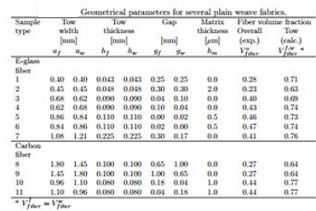Calculate the tow fiber volume fraction

Response to the following problem:

Recalculate the tow elastic properties E1, E2, G12, G23, ν12, ν23 of weave type 1 in Table using the values of V tow f iber calculated in Problem 1.

Problem 1:  Calculate the mesoscale tow volume fraction Vmeso and the tow fiber volume fraction V f f iber = V w f iber of the weave type 5 in Table , based on the information given in the table.